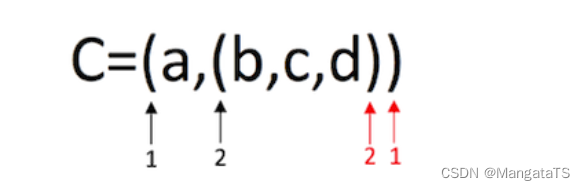# 考研数据结构与算法（五）数组¶

## 二、二维数组的存储方式¶

### 2.1 按行优先¶

$$Loc_{a[i][j]} = Loc_{a} + [i\times n + j] \times L$$

### 2.2 按列优先¶

$$Loc_{a[i][j]} = Loc_{a} + [j\times n + i] \times L$$

## 三、特殊矩阵和压缩矩阵¶

### 3.1 特殊矩阵¶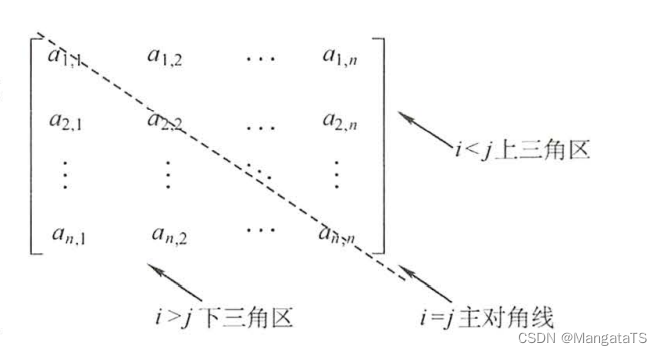#### 3.1.1 对称矩阵¶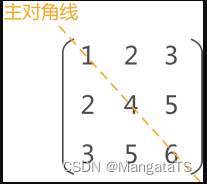$k = \begin{cases} \frac{i(i-1)}{2}+j-1, & \text {i>=j，下三角区} \\ \frac{j(j-1)}{2}+i-1, &\text{i<j，上三角区} \end{cases}$

#### 3.1.2 上（下）三角矩阵¶

$k = \begin{cases} \frac{i(i-1)}{2}+j-1, & \text {i>=j，下三角区} \\ \frac{n(n+1)}{2}, &\text{i<j，上三角区} \end{cases}$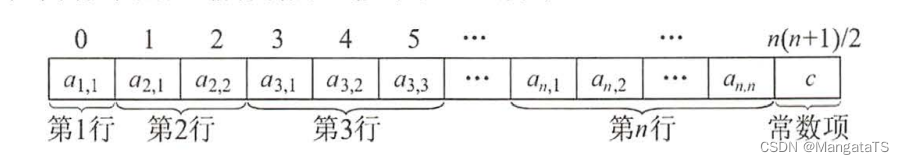$k = \begin{cases} \frac{i(2n-i+1)}{2}-n+j - 1, & \text {i<=j，上三角区} \\ \frac{n(n+1)}{2}, &\text{i<j，下三角区} \end{cases}$

#### 3.1.3 稀疏矩阵¶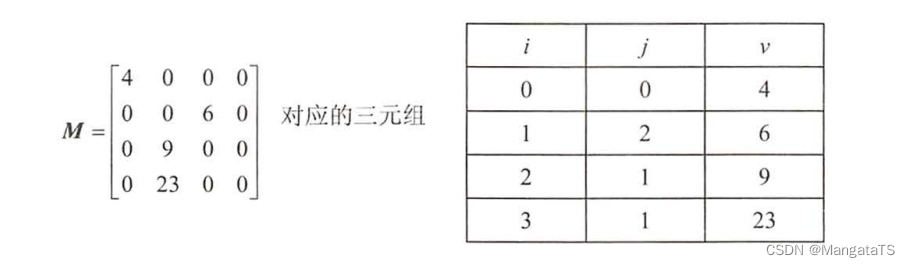#### 3.1.4 三对角矩阵¶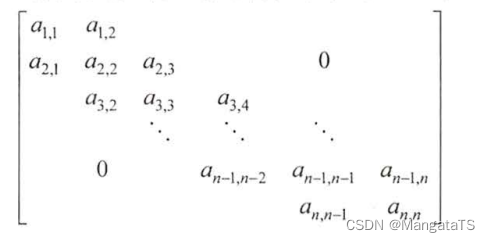$$k = 2i + j - 3$$$$i = \left \lfloor (k+1)/3 + 1 \right \rfloor,j = k - 2i + 3$$ 例如，当 $$k$$ 等于 $$0$$ 的时候，$$i = \left \lfloor (0+1)/3 + 1 \right \rfloor = 1，j = 0-2\times 1 + 3 = 1$$ 即第一个位置存放的是矩阵元素 $$a$$

## 四、广义表的定义和存储结构¶

### 4.1 广义表的定义¶

广义表，又称列表，也是一种线性存储结构，既可以存储不可再分的元素，也可以存储广义表，记作：$$LS = (a_1,a_2,…,a_n)$$ ，其中，LS 代表广义表的名称，$$a_n$$ 表示广义表存储的数据，广义表中每个 $$a_i$$ 既可以代表 单个元素 ，也可以代表另一个 广义表 ,分别称为广义表 $$LS$$原子子表

### 4.2 广义表的存储结构¶

typedef enum{ATOM,LIST}ElemTag;//ATOM=0表示原子结点，ATOM=1表示子表
typedef struct GLNode{
ElemTag tag;
union {
ATomType atom;
struct {struct GLNode *hp,*tp} ptr;
};
}*Glist;


### 4.3 广义表的深度和长度¶

• 广义表的长度，指的是广义表中所包含的数据元素的个数

• 计算元素个数时，广义表中存储的每个原子算作一个数据，同样每个子表也只算作是一个数据

• $$LS = {a_1,a_2,…,a_n}$$ 的长度为 $$n$$
• 广义表 $${a,{b,c,d}}$$ 的长度为 2；
• 广义表 $${{a,b,c}}$$ 的长度为 1；
• 空表 $${}$$ 的长度为 0。